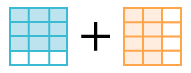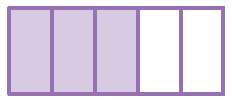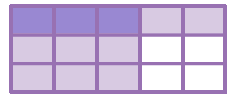### Home > ACC6 > Chapter 3 Unit 11 > Lesson CC2: 3.2.1 > Problem3-38

3-38.

Simplify the following fraction expressions. Show all of your work.

1. $\frac{3}{4}+\frac{2}{3}$Draw diagrams to represent each fraction. Diagram expression with 2 equal sized rectangles: Left Rectangle + Right Rectangle. Rectangle A, 4 rows, with 3 shaded. Rectangle B, 3 columns, with 2 shaded.

Now change the diagram so that each whole is divided into equal portions. Changes: Left rectangle, divided into 3 columns, 9 out of 12 shaded. Right column, divided into 4 rows, 8 out of 12 shaded.

1. $\frac{7}{8}-\frac{1}{4}$

Follow the steps in part (a) to solve the problem.

1. $\frac{3}{5}\cdot\frac{1}{3}$

Draw a rectangle.Shade in three fifths. Rectangle divided vertically into 5 columns, with 3 shaded.

Shade in one third in the other direction. Changes: Rectangle, divided into 3 rows, with first row shaded. The first 3 cells in the first row are shaded twice.

1. $\frac{4}{7}\cdot\frac{2}{3}$
$\frac{8}{21}$